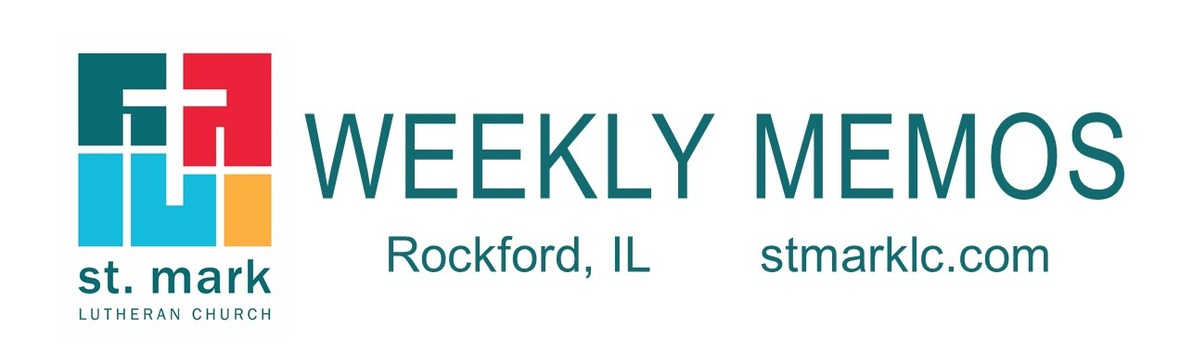table div table+table div table{width:100%;padding:0}table div table+table div table img{width:96.23%;padding:0;float:none}table div table+table div table td{width:100%;padding:0 1.88% 18px}/* styles */## Pastoral Reflection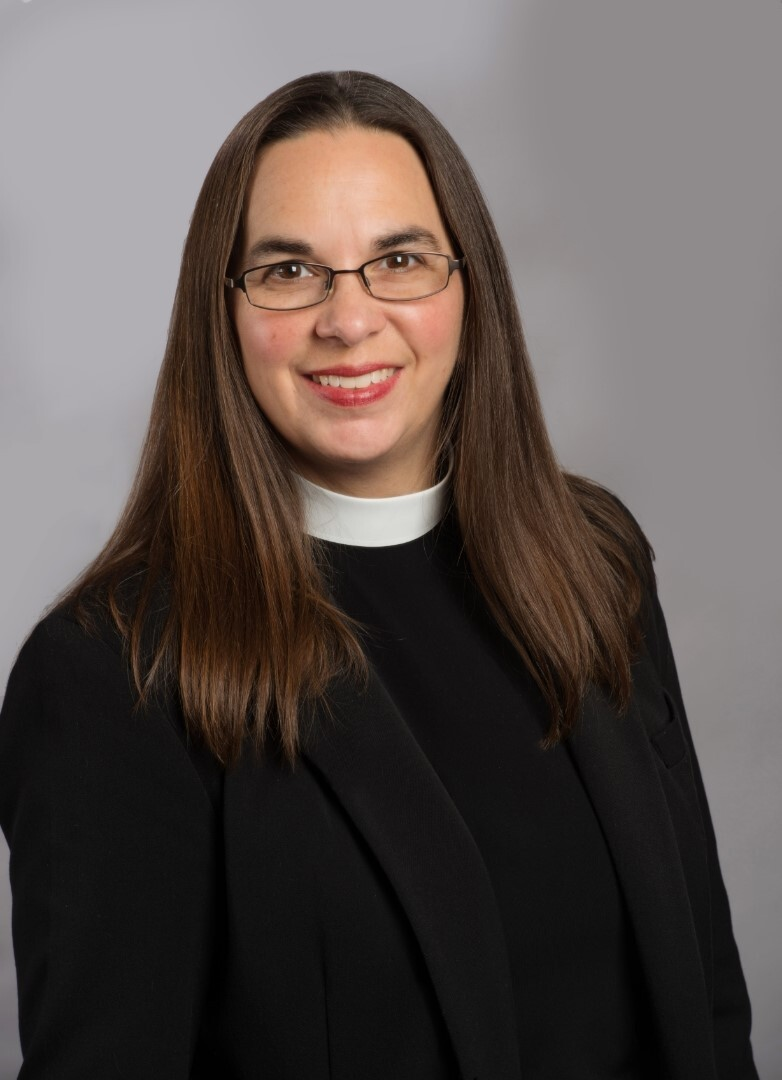Oh, the joy!
Pastor Katrina Steingraeber

What an amazing and energetic day! I was filled with joy as we worshiped together and gathered together on September 10th. SeptemberFest was so fun!

 table div table+table+table+table+table div table{width:100%;padding:0}table div table+table+table+table+table div table img{width:96.23%;padding:0;float:none}table div table+table+table+table+table div table td{width:100%;padding:0 1.88% 18px}/* styles */## This Week at St. Mark September 17 - 24, 2023

 table div table+table+table+table+table+table+table div table{width:100%;padding:0}table div table+table+table+table+table+table+table div table img{width:96.23%;padding:0;float:none}table div table+table+table+table+table+table+table div table td{width:100%;padding:0 1.88% 18px}/* styles */## Sunday, September 17

9:00 A.M. Worship with Live-Stream
9:00 A.M. All first-year confirmation students, 3-year-olds, & 3rd graders will receive a Bible & Sunday School Teacher Installation
10:00 A.M. Fellowship Hour with coffee and donuts
10:05 A.M. Sunday School Begins
11:00 A.M. Worship in Fellowship Hall
6:30 P.M. Youth Group

## Monday, September 18

10:30 A.M. Mosaic Hub
10:30 A.M. Classic Chorale Rehearses
6:30 P.M. Emotions Anonymous

## Tuesday, September 19

10:00 A.M. St. Mark Duplicate Bridge
4:00 P.M. Endowment Meeting
7:00 P.M. Executive Meeting

## Wednesday, September 20

9:00 A.M. Women's Bible Study
9:30 A.M. St. Mark Ringers
6:30 P.M. Confirmation Begins
7:00 P.M. Voices of St. Mark

## Thursday, September 21

9:00 A.M. Cherry Valley Bridge Group
6:30 P.M. Emotions Anonymous
6:30 P.M. Blue Lights

## Friday, September 22

7:30 A.M. St. Mark Breakfast Group at Denny's Restaurant

## Sunday, September 24

9:00 A.M. Worship with Live-Stream
10:00 A.M. Fellowship Hour with coffee and donuts
10:05 A.M. Sunday School Begins
11:00 A.M. Worship in Fellowship Hall
6:30 P.M. Youth Group

 table div table+table+table+table+table+table+table+table+table div table{width:100%;padding:0}table div table+table+table+table+table+table+table+table+table div table img{width:96.23%;padding:0;float:none}table div table+table+table+table+table+table+table+table+table div table td{width:100%;padding:0 1.88% 18px}/* styles */## News and Events

 table div table+table+table+table+table+table+table+table+table+table+table div table{width:100%;padding:0}table div table+table+table+table+table+table+table+table+table+table+table div table img{width:96.23%;padding:0;float:none}table div table+table+table+table+table+table+table+table+table+table+table div table td{width:100%;padding:0 1.88% 18px}/* styles *//* styles */ Join us this Sunday for the 16th Sunday after Pentecost, September 17 9:00 a.m. - Traditional Worship in the Sanctuary- Prelude will start at 8:55. 10:00 A.M. Fellowship with coffee and donuts in the cafe only 10:05 A.M. Sunday School 10:05 A.M. Adult Forum 11:00 A.M. Praise Service 6:30 P.M. Youth Group at St. Mark If you are unable to be here, click here to join us at 8:55 for our live-stream service.
 table div table+table+table+table+table+table+table+table+table+table+table+table+table div table{width:100%;padding:0}table div table+table+table+table+table+table+table+table+table+table+table+table+table div table img{width:96.23%;padding:0;float:none}table div table+table+table+table+table+table+table+table+table+table+table+table+table div table td{width:100%;padding:0 1.88% 18px}/* styles */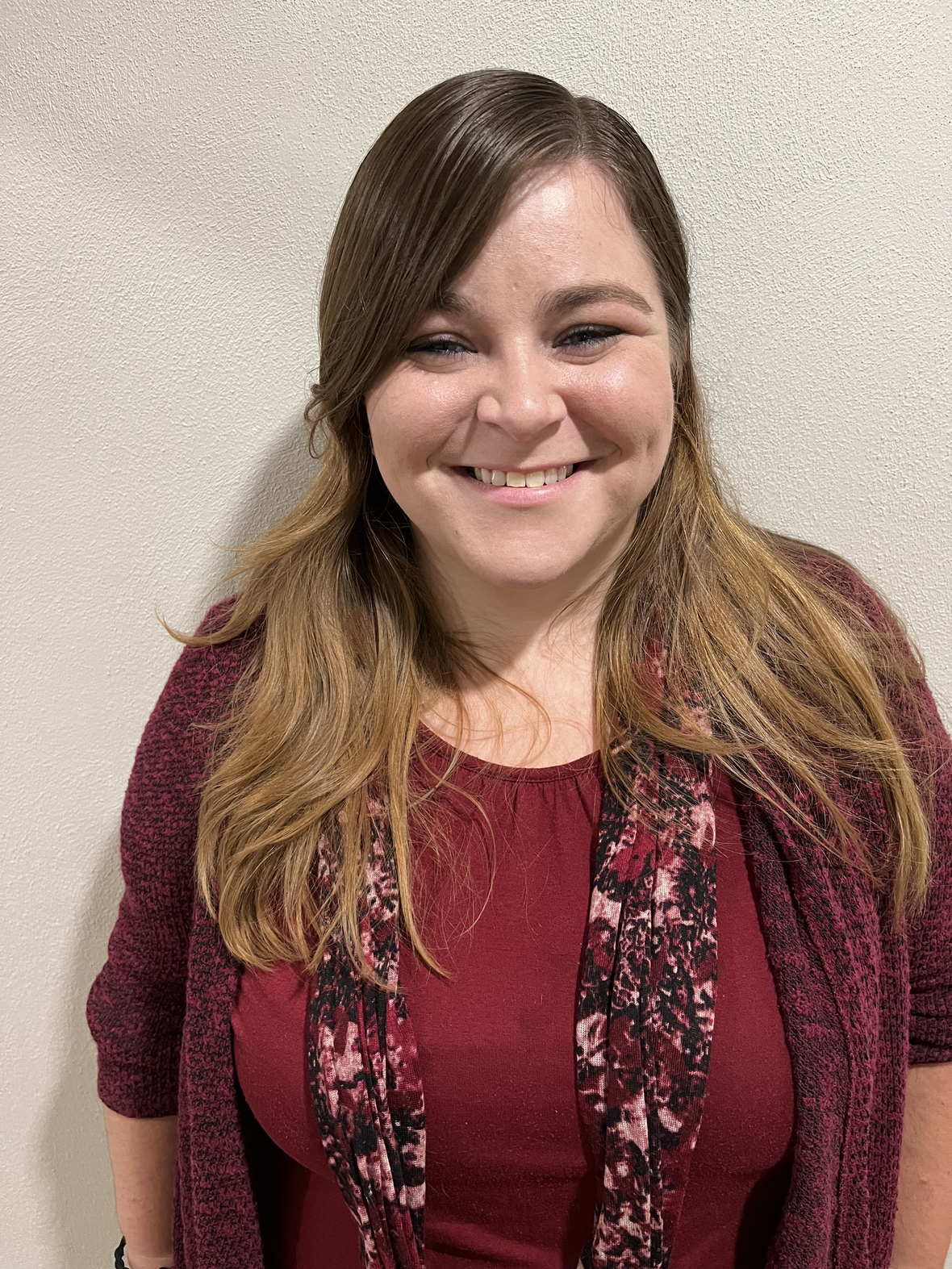Welcome Cassie!
We are so excited to announce that Cassie Boyer has joined our staff as our new Shared Youth Ministry Director! As part of our innovative partnership with Grace Lutheran Church, Cassie will be leading a unified youth ministry program for the middle school and high school students of both congregations. As we welcome her with open arms, we also give thanks to everyone who aided in our discernment and search process.

Hello! My name is Cassie, short for Cassandra. I was born in Rockford at the old Rockford Memorial Hospital, grew up in the Rockford/ Loves Park area, and graduated from Harlem High School. I love working with youth and am slowly working towards getting my bachelor’s degree in Middle Grades Education, from Rockford University, with an emphasis in Alternative Education (education that takes place outside of a typical classroom setting). In my spare time, I love to spend time with my sister and dog, do random science experiments, write poetry and short stories, and do arts and crafts. Last year I went to Ecuador with my school, taught science in a one-room schoolhouse, and helped to build a playground for a small village there. I can’t wait to meet you in person and get to know more about you! - Cassie B

 table div table+table+table+table+table+table+table+table+table+table+table+table+table+table+table div table{width:100%;padding:0}table div table+table+table+table+table+table+table+table+table+table+table+table+table+table+table div table img{width:96.23%;padding:0;float:none}table div table+table+table+table+table+table+table+table+table+table+table+table+table+table+table div table td{width:100%;padding:0 1.88% 18px}/* styles *//* styles */ St. Mark SeptemberFest Outreach Service Projects Continues through September 17 Thank you for the generous donations of cereal and luggage. These donations are greatly appreciated. The two service projects for SeptemberFest will continue through September 17th. The Back to School Cereal Drive for the Rock River Valley Pantry and the luggage, duffel bags, and adult-sized backpacks for LSSI foster children. There are barrels in the narthex to collect cereal boxes of any kind or size. The luggage can be placed in or near the red truck, please donate luggage that is appropriately sized for children and teens. Monetary donations are welcomed, if writing a check, please note in that the money is for the Cereal Drive. You may also make a donation here through push pay. Both of these are a great opportunity to serve our neighbors in need.
 table div table+table+table+table+table+table+table+table+table+table+table+table+table+table+table+table+table div table{width:100%;padding:0}table div table+table+table+table+table+table+table+table+table+table+table+table+table+table+table+table+table div table img{width:96.23%;padding:0;float:none}table div table+table+table+table+table+table+table+table+table+table+table+table+table+table+table+table+table div table td{width:100%;padding:0 1.88% 18px}/* styles *//* styles */ SeptemberFest was a Blast! Here is the link to the service if you couldn't make it.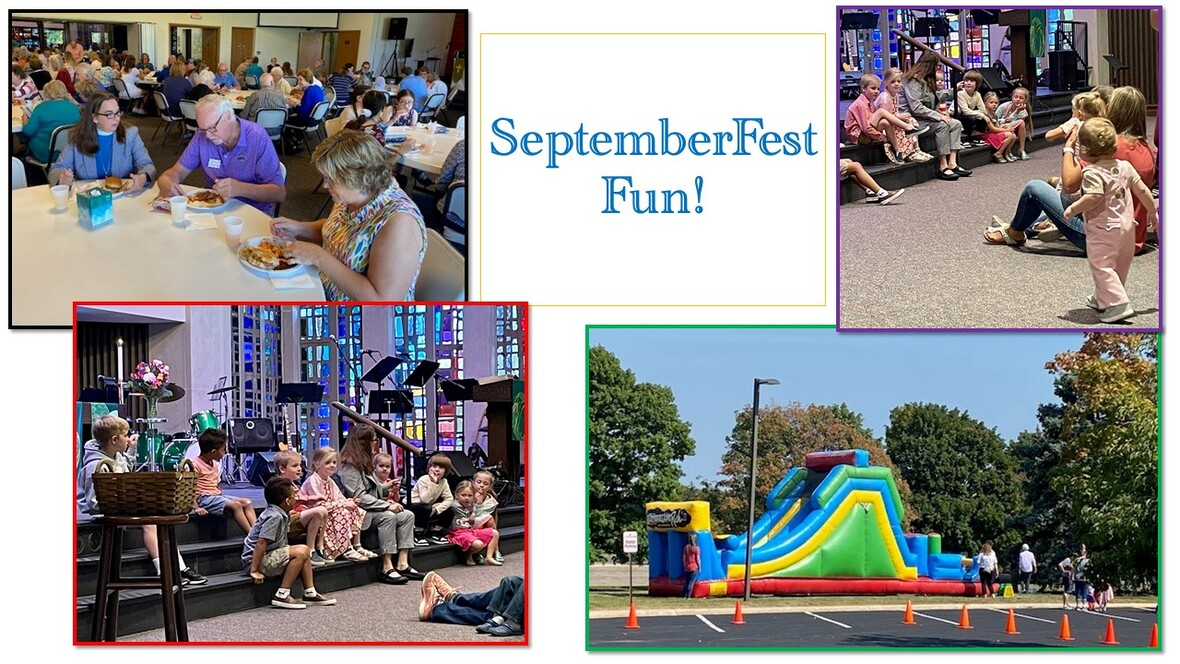table div table+table+table+table+table+table+table+table+table+table+table+table+table+table+table+table+table+table+table+table div table{width:100%;padding:0}table div table+table+table+table+table+table+table+table+table+table+table+table+table+table+table+table+table+table+table+table div table img{width:96.23%;padding:0;float:none}table div table+table+table+table+table+table+table+table+table+table+table+table+table+table+table+table+table+table+table+table div table td{width:100%;padding:0 1.88% 18px}/* styles */Sunday School Begins Sunday!
Don't forget, Sunday School begins this Sunday at 10:05 in the downstairs classrooms. We will first gather as a large group for an introduction to the week's Bible story, then move to age-level classes for lesson activities.

If you still need to register click on this link. Sunday School Sign-up Form

 table div table+table+table+table+table+table+table+table+table+table+table+table+table+table+table+table+table+table+table+table+table+table div table{width:100%;padding:0}table div table+table+table+table+table+table+table+table+table+table+table+table+table+table+table+table+table+table+table+table+table+table div table img{width:96.23%;padding:0;float:none}table div table+table+table+table+table+table+table+table+table+table+table+table+table+table+table+table+table+table+table+table+table+table div table td{width:100%;padding:0 1.88% 18px}/* styles */St. Mark "Makers"
St. Mark "makers" will meet on Monday, October 2 from 2:00 - 4:00 P.M. Come get your craft on st St. Mark! Watch for details of our next project. Sign-up starts soon! Friends welcome!

Below are pictures of the finished projects from the September event. The yarn pumpkins and painted acorns. Welcome Fall!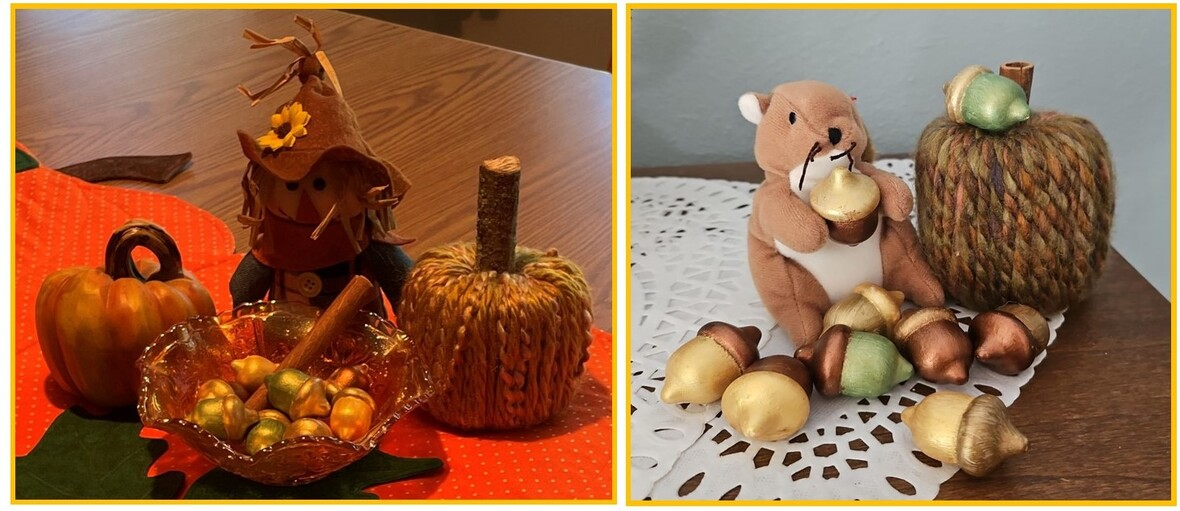table div table+table+table+table+table+table+table+table+table+table+table+table+table+table+table+table+table+table+table+table+table+table+table+table+table div table{width:100%;padding:0}table div table+table+table+table+table+table+table+table+table+table+table+table+table+table+table+table+table+table+table+table+table+table+table+table+table div table img{width:96.23%;padding:0;float:none}table div table+table+table+table+table+table+table+table+table+table+table+table+table+table+table+table+table+table+table+table+table+table+table+table+table div table td{width:100%;padding:0 1.88% 18px}/* styles */Confirmation
Regular Confirmation classes will begin on September 20th and we will meet from 6:30 pm to 8 pm. If you have any questions, please contact Pastor Katrina at prkatrina@stmarklc.com.

 /* styles */
 table div table+table+table+table+table+table+table+table+table+table+table+table+table+table+table+table+table+table+table+table+table+table+table+table+table+table+table+table div table{width:100%;padding:0}table div table+table+table+table+table+table+table+table+table+table+table+table+table+table+table+table+table+table+table+table+table+table+table+table+table+table+table+table div table img{width:96.23%;padding:0;float:none}table div table+table+table+table+table+table+table+table+table+table+table+table+table+table+table+table+table+table+table+table+table+table+table+table+table+table+table+table div table td{width:100%;padding:0 1.88% 18px}/* styles */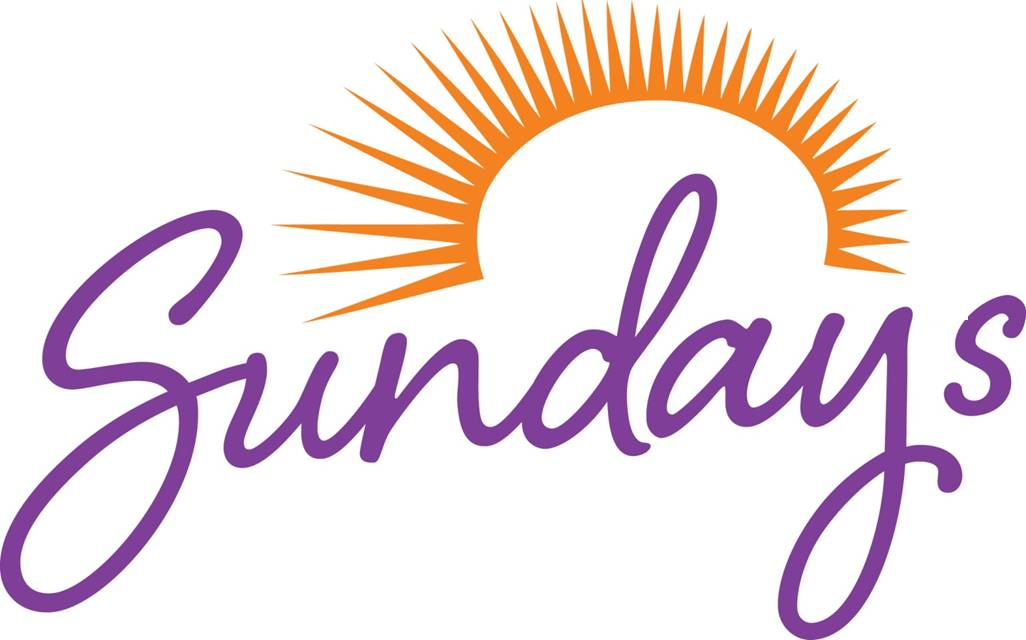Our Sunday morning Adult Forum is during the learning hour at 10:05 am in the Adult Ministry room. Sunday School and Fellowship also occur during this time between services. Bring your donut and coffee and join us. The Adult Forum will change from week to week, and you can expect to see opportunities for Bible studies, book studies, presentations from local agencies, and group discussions.

 table div table+table+table+table+table+table+table+table+table+table+table+table+table+table+table+table+table+table+table+table+table+table+table+table+table+table+table+table+table+table div table{width:100%;padding:0}table div table+table+table+table+table+table+table+table+table+table+table+table+table+table+table+table+table+table+table+table+table+table+table+table+table+table+table+table+table+table div table img{width:96.23%;padding:0;float:none}table div table+table+table+table+table+table+table+table+table+table+table+table+table+table+table+table+table+table+table+table+table+table+table+table+table+table+table+table+table+table div table td{width:100%;padding:0 1.88% 18px}/* styles */St. Mark Women's Bible Study
The Bible Study will resume weekly meetings on September 20th at 9:00 a.m. in the Conference Room next to the Cafe. This year the group will read and discuss Rev. Dr. Wayne Viereck's book entitled Christian Faith in the 21st Century. After the completion of the book, we will read and study the book of Romans. The Bible Study focuses on God's Word through Scripture readings, discussion, personal experiences, and fellowship. If you have any questions please let me know at dsiverson5@gmail.com or 815-218-5152 (Deb Iverson).

 table div table+table+table+table+table+table+table+table+table+table+table+table+table+table+table+table+table+table+table+table+table+table+table+table+table+table+table+table+table+table+table+table div table{width:100%;padding:0}table div table+table+table+table+table+table+table+table+table+table+table+table+table+table+table+table+table+table+table+table+table+table+table+table+table+table+table+table+table+table+table+table div table img{width:96.23%;padding:0;float:none}table div table+table+table+table+table+table+table+table+table+table+table+table+table+table+table+table+table+table+table+table+table+table+table+table+table+table+table+table+table+table+table+table div table td{width:100%;padding:0 1.88% 18px}/* styles */Men's Bible Study
The Men’s Bible Study will begin on September 27th. Please note the start date has changed from the 20th to the 27th. We will meet at 9:00 am in the Café. We are using the last book in the Animate Faith Series titled Practices. Please let Pastor Katrina know if you plan to join the Men’s Bible Study, to make sure there are enough books ordered for everyone. If you have any questions, please email Pastor Katrina at prkatrina@stmarklc.com.

 table div table+table+table+table+table+table+table+table+table+table+table+table+table+table+table+table+table+table+table+table+table+table+table+table+table+table+table+table+table+table+table+table+table+table div table{width:100%;padding:0}table div table+table+table+table+table+table+table+table+table+table+table+table+table+table+table+table+table+table+table+table+table+table+table+table+table+table+table+table+table+table+table+table+table+table div table img{width:96.23%;padding:0;float:none}table div table+table+table+table+table+table+table+table+table+table+table+table+table+table+table+table+table+table+table+table+table+table+table+table+table+table+table+table+table+table+table+table+table+table div table td{width:100%;padding:0 1.88% 18px}/* styles */The Happy Campers(formerly Empty Nesters) have invited Gary Anderson to speak to our group. Gary is an architect, an advocate of historic preservation, and an ambassador of Rockford. You may have participated in a bike or walking tour with Gary. Gary is well known for his tireless effort to preserve historic buildings in Rockford and beyond. Gary will present right here at St. Mark. Come help us kick-off a fun and informative social event. Join us Thursday, October 5, 5:00-6:30 P.M. At St. Mark. Friends are welcome!

There will be light refreshments and time for discussion and questions with Gary. Please sign-up between services and say you will join us. You may also call and sign-up through the church office by October 1.

 table div table+table+table+table+table+table+table+table+table+table+table+table+table+table+table+table+table+table+table+table+table+table+table+table+table+table+table+table+table+table+table+table+table+table+table+table div table{width:100%;padding:0}table div table+table+table+table+table+table+table+table+table+table+table+table+table+table+table+table+table+table+table+table+table+table+table+table+table+table+table+table+table+table+table+table+table+table+table+table div table img{width:96.23%;padding:0;float:none}table div table+table+table+table+table+table+table+table+table+table+table+table+table+table+table+table+table+table+table+table+table+table+table+table+table+table+table+table+table+table+table+table+table+table+table+table div table td{width:100%;padding:0 1.88% 18px}/* styles */## Outreach Opportunities

 table div table+table+table+table+table+table+table+table+table+table+table+table+table+table+table+table+table+table+table+table+table+table+table+table+table+table+table+table+table+table+table+table+table+table+table+table+table+table div table{width:100%;padding:0}table div table+table+table+table+table+table+table+table+table+table+table+table+table+table+table+table+table+table+table+table+table+table+table+table+table+table+table+table+table+table+table+table+table+table+table+table+table+table div table img{width:96.23%;padding:0;float:none}table div table+table+table+table+table+table+table+table+table+table+table+table+table+table+table+table+table+table+table+table+table+table+table+table+table+table+table+table+table+table+table+table+table+table+table+table+table+table div table td{width:100%;padding:0 1.88% 18px}/* styles */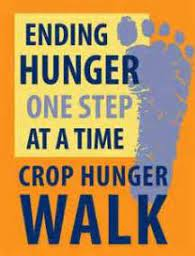CROP Hunger Walk
CROP Hunger Walk is October 15th, 2023 and it begins at 1 pm at the Riverfront Museum Park, 711 N. Main Street. If you are interested in participating in the CROP Hunger Walk, please sign up at www.crophungerwalk.org/rockfordil. If you are unable to participate you can donate to CROP Hunger Walk by writing a check and putting in the memo for CROP Walk. There will be a link in Push Pay as well.

 table div table+table+table+table+table+table+table+table+table+table+table+table+table+table+table+table+table+table+table+table+table+table+table+table+table+table+table+table+table+table+table+table+table+table+table+table+table+table+table+table div table{width:100%;padding:0}table div table+table+table+table+table+table+table+table+table+table+table+table+table+table+table+table+table+table+table+table+table+table+table+table+table+table+table+table+table+table+table+table+table+table+table+table+table+table+table+table div table img{width:96.23%;padding:0;float:none}table div table+table+table+table+table+table+table+table+table+table+table+table+table+table+table+table+table+table+table+table+table+table+table+table+table+table+table+table+table+table+table+table+table+table+table+table+table+table+table+table div table td{width:100%;padding:0 1.88% 18px}/* styles *//* styles */ Becca's Closet is located at Our Savior's Church at 3300 Rural Street.
 table div table+table+table+table+table+table+table+table+table+table+table+table+table+table+table+table+table+table+table+table+table+table+table+table+table+table+table+table+table+table+table+table+table+table+table+table+table+table+table+table+table+table+table div table{width:100%;padding:0}table div table+table+table+table+table+table+table+table+table+table+table+table+table+table+table+table+table+table+table+table+table+table+table+table+table+table+table+table+table+table+table+table+table+table+table+table+table+table+table+table+table+table+table div table img{width:96.23%;padding:0;float:none}table div table+table+table+table+table+table+table+table+table+table+table+table+table+table+table+table+table+table+table+table+table+table+table+table+table+table+table+table+table+table+table+table+table+table+table+table+table+table+table+table+table+table+table div table td{width:100%;padding:0 1.88% 18px}/* styles */Rockford 1 Million Meal Pack
Help turn hunger into hope by packing meals for children in need in Zambia, Ecuador, and the Dominican Republic on Saturday, Sept. 23, and Sunday Sept. 24 at the UW Health Sports Factory. Go to katw.org/rockford1m to sign up to pack meals.

 table div table+table+table+table+table+table+table+table+table+table+table+table+table+table+table+table+table+table+table+table+table+table+table+table+table+table+table+table+table+table+table+table+table+table+table+table+table+table+table+table+table+table+table+table+table div table{width:100%;padding:0}table div table+table+table+table+table+table+table+table+table+table+table+table+table+table+table+table+table+table+table+table+table+table+table+table+table+table+table+table+table+table+table+table+table+table+table+table+table+table+table+table+table+table+table+table+table div table img{width:96.23%;padding:0;float:none}table div table+table+table+table+table+table+table+table+table+table+table+table+table+table+table+table+table+table+table+table+table+table+table+table+table+table+table+table+table+table+table+table+table+table+table+table+table+table+table+table+table+table+table+table+table div table td{width:100%;padding:0 1.88% 18px}/* styles */## Youth MinistryIt is time for the Youth Group to begin. We will meet on two Sundays in September. The plan is to meet 2 or 3 Sundays a month. We will gather in the Café from 6:30 pm to 8 pm to play games, catch up, or just hang out. If you would like to go and do something, please bring your ideas on September 17th. We have one outing planned for October. We are excited to start the fall youth group and hope to see you all!

September 17th Youth Group:
Come to St. Mark at 6:30 pm on Sunday to meet our new Youth Ministry Director Cassie Boyer. This is an opportunity for you all to spend time getting to know Cassie and a time for her to get to know you all. Please join me in welcoming Cassie.

 /* styles */ St. Mark Youth Group September and October Schedules: September 17th youth group will meet with Pastor Katrina. * September 24th youth group will meet with Pastor Chad. * October 8th TBD either a 2nd Sunday gathering or a St. Mark youth group gathering. * October 15th St. Mark youth group will go to Edwards Apple Orchard 7061 Centerville Road, Popular Grove, Illinois 61065. We will meet at Edwards from 2:30pm until 4pm. If you need a ride to the apple orchard, please let Pastor Katrina know. * October 22nd St. Mark youth group.
 table div table+table+table+table+table+table+table+table+table+table+table+table+table+table+table+table+table+table+table+table+table+table+table+table+table+table+table+table+table+table+table+table+table+table+table+table+table+table+table+table+table+table+table+table+table+table+table+table+table div table{width:100%;padding:0}table div table+table+table+table+table+table+table+table+table+table+table+table+table+table+table+table+table+table+table+table+table+table+table+table+table+table+table+table+table+table+table+table+table+table+table+table+table+table+table+table+table+table+table+table+table+table+table+table+table div table img{width:96.23%;padding:0;float:none}table div table+table+table+table+table+table+table+table+table+table+table+table+table+table+table+table+table+table+table+table+table+table+table+table+table+table+table+table+table+table+table+table+table+table+table+table+table+table+table+table+table+table+table+table+table+table+table+table+table div table td{width:100%;padding:0 1.88% 18px}/* styles */## Remember In Our Prayers

 table div table+table+table+table+table+table+table+table+table+table+table+table+table+table+table+table+table+table+table+table+table+table+table+table+table+table+table+table+table+table+table+table+table+table+table+table+table+table+table+table+table+table+table+table+table+table+table+table+table+table+table div table{width:100%;padding:0}table div table+table+table+table+table+table+table+table+table+table+table+table+table+table+table+table+table+table+table+table+table+table+table+table+table+table+table+table+table+table+table+table+table+table+table+table+table+table+table+table+table+table+table+table+table+table+table+table+table+table+table div table img{width:96.23%;padding:0;float:none}table div table+table+table+table+table+table+table+table+table+table+table+table+table+table+table+table+table+table+table+table+table+table+table+table+table+table+table+table+table+table+table+table+table+table+table+table+table+table+table+table+table+table+table+table+table+table+table+table+table+table+table div table td{width:100%;padding:0 1.88% 18px}/* styles */## Our St. Mark Members:

Lis Pearson, Joe Garcea, Jeanne Zeschke, Ulla Kercher, Mark Honzel, Lorine Kortus, Beth Johnson, Carol Kilts, Fritzi Panjkovich, Jan Cotter, Diane Swanson, Sherri Lindquist, Bette Patterson.

## Family and Friends of St. Mark Members:

Kyle Larson, Ruth Hewitt, Avis Holmes, Mike Balles, Chris Nack, Ron Gentry, Terry Backen, John Ayotte, Carrie Kielty, Ed Sobczyk, Elizabeth Woodworth, Carrie Nelson, Bill Hart, Ellie Krepfle, Tom Montgomery, Jon Baylander, Jenny Hobby, Bill King, Carolyn King.

## Those serving in the military and their families:

Logan Gehrke, Hunter Haggestad, Scot Hornick, Jim Tammen, Brandon Ballenger, Taylor Eversole.

Strengthen and encourage those in public health services and in the medical profession: care-givers, nurses, attendants, doctors, all who commit themselves to care for the sick and their families.

 table div table+table+table+table+table+table+table+table+table+table+table+table+table+table+table+table+table+table+table+table+table+table+table+table+table+table+table+table+table+table+table+table+table+table+table+table+table+table+table+table+table+table+table+table+table+table+table+table+table+table+table+table+table div table{width:100%;padding:0}table div table+table+table+table+table+table+table+table+table+table+table+table+table+table+table+table+table+table+table+table+table+table+table+table+table+table+table+table+table+table+table+table+table+table+table+table+table+table+table+table+table+table+table+table+table+table+table+table+table+table+table+table+table div table img{width:96.23%;padding:0;float:none}table div table+table+table+table+table+table+table+table+table+table+table+table+table+table+table+table+table+table+table+table+table+table+table+table+table+table+table+table+table+table+table+table+table+table+table+table+table+table+table+table+table+table+table+table+table+table+table+table+table+table+table+table+table div table td{width:100%;padding:0 1.88% 18px}/* styles *//* styles */ Office Hours Monday through Thursday 9:00 A.M. - 4:00 P.M. Friday - 9:00 A.M. - 1:00 P.M. Telephone: 815-398-3557 Publications St. Mark Memos, the weekly e-newsletter, is distributed each Thursday. The deadline for submission is Monday by 3:00 P.M. Please click to send submissions. Worship Services Miss a Sunday at St. Mark? Please visit our website and watch the service online. Please click to watch.
 table div table+table+table+table+table+table+table+table+table+table+table+table+table+table+table+table+table+table+table+table+table+table+table+table+table+table+table+table+table+table+table+table+table+table+table+table+table+table+table+table+table+table+table+table+table+table+table+table+table+table+table+table+table+table+table div table{width:100%;padding:0}table div table+table+table+table+table+table+table+table+table+table+table+table+table+table+table+table+table+table+table+table+table+table+table+table+table+table+table+table+table+table+table+table+table+table+table+table+table+table+table+table+table+table+table+table+table+table+table+table+table+table+table+table+table+table+table div table img{width:96.23%;padding:0;float:none}table div table+table+table+table+table+table+table+table+table+table+table+table+table+table+table+table+table+table+table+table+table+table+table+table+table+table+table+table+table+table+table+table+table+table+table+table+table+table+table+table+table+table+table+table+table+table+table+table+table+table+table+table+table+table+table div table td{width:100%;padding:0 1.88% 18px}/* styles */